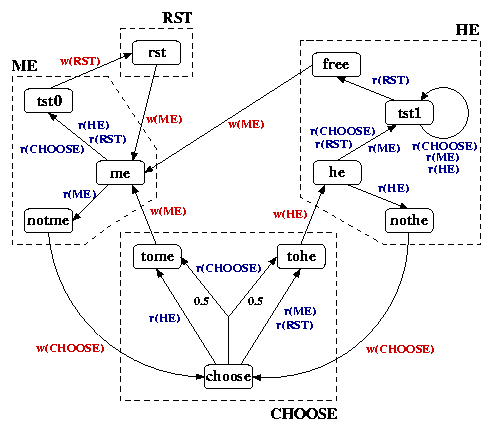# Randomised Two Process Wait-Free Test-and-Set

(Tromp and Vitanyi)

### Introduction

This case study considers the algorithm of Tromp and Vitanyi [TV02], the first explicit randomised algorithm for two-process wait-free test-and-set.

The protocol is used to select a unique process. It is designed for two processes, P0 and P1, acting concurrently and involves two four valued single writer/single reader atomic variables R0 and R1. Process Pi only writes to variable Ri and only reads variable R(1-i). Hence, the reads and the writes in the protocol do not need to be parametrised by the variable they access. The protocol for a single process is given below and the transitions are labelled by reads (r(VALUE)) and writes (w(VALUE)).Note that there is a probabilistic choice in state choose when the process also reads value CHOOSE. The states enclosed within the dotted lines correspond to the set of states in which the processes own shared variable has the same value. The initial state is rst, and the states tst0 and tst1 correspond to the completing the test-and-set operation with success and failure respectively.

### The PRISM Model

The PRISM code for this protocol is given below. Note that the description also includes a tester processes which is required in the model checking phase.

```// randomized two process wait-free test-and-set [TV02]
// gxn/dxp 12/07/02

mdp

// tester process
module tester

t : [0..1];

[] t=0 -> (t'=1); // start test

endmodule

// process 0
module proc0

// local states
l0 : [0..10];
// 0  - rst
// 1  - me
// 2  - not me
// 3  - choose
// 4  - to me
// 5  - to he
// 6  - he
// 7  - not he
// 8  - tst0
// 9  - tst1
// 10 - free

// shared variable owned by process 0
R0 : [0..3];
// 0 - rst
// 1 - choose
// 2 - he
// 3 - me

// rst
[p0] l0=0 -> (R0'=3) & (l0'=1);
// me
[p0] l0=1 & R1=3  -> (l0'=2);
[p0] l0=1 & !R1=3 -> (l0'=8); // finished and test not started
// not me
[p0] l0=2 -> (R0'=1) & (l0'=3);
// choose
[p0] l0=3 & R1=2 -> (l0'=4); // R1=he
[p0] l0=3 & R1=1 -> 0.5 : (l0'=4) + 0.5 : (l0'=5);  // R1=choose
[p0] l0=3 & !(R1=2 | R1=1) -> (l0'=5); // otherwise
// to me
[p0] l0=4 -> (R0'=3) & (l0'=1);
// to he
[p0] l0=5 -> (R0'=2) & (l0'=6);
// he
[p0] l0=6 & R1=2  -> (l0'=7);
[p0] l0=6 & !R1=2 -> (l0'=9); // finished and test not started
// not he
[p0] l0=7 -> (R0'=1) & (l0'=3);
// tst0 (removed transitions once test starts as only looking at one test and set operation)
[p0] t=0 & l0=8 -> (R0'=0) & (l0'=0);
// tst1 (removed transitions once test starts as only looking at one test and set operation)
[p0] t=0 & l0=9 & !R1=0 -> (l0'=9); // R1 not rst
[p0] t=0 & l0=9 & R1=0 -> (l0'=10); // R1 equals rst
// free
[p0] l0=10 -> (R0'=3) & (l0'=1);

endmodule

// construct process 1 through renaming
module proc1=proc0[l0=l1,R0=R1,R1=R0,p0=p1] endmodule

// rewards
rewards "process0" // reads/writes by process 0 (all transitions of process 0 require one access)
[p0] t=1 : 1;
endrewards
rewards "process1" // reads/writes by process 1 (all transitions of process 1 require one access)
[p1] t=1 : 1;
endrewards
```

It took 0.03 to construct the model, the model has 196 states, and it required 19 iterations to find these reachable states.

### Model Checking

We showed that the protocol is wait free, by verifying that, under any scheduling, the maximum expected number of accesses by one process to shared variables during the execution of a test-and-set operation is less than or equal to 10.

More precisely we check that the following PCTL specifications hold in all states.

```// maximum expected operations for one test and set operation is less than 10
filter(forall, t=1 => R{"process0"}<=10 [ F t=1 & (l0=8 | l0=9) ])
filter(forall, t=1 => R{"process1"}<=10 [ F t=1 & (l1=8 | l1=9) ])

// display actual values for process 0 (once test starts)
R{"process0"}max=? [ F t=1 & (l0=8 | l0=9) {t=1} ]
```